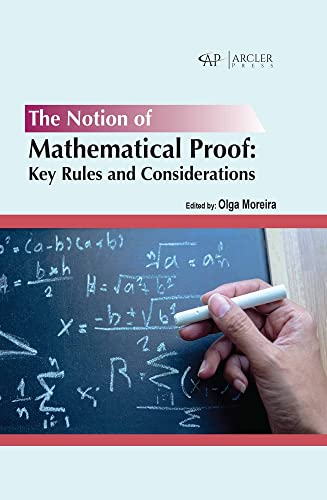# The notion of mathematical proof: Key rules and considerations

• Length: 424 pages
• Edition: 1
• Publisher:
• Publication Date: 2022-12-01
• ISBN-10: 1774694980
• ISBN-13: 9781774694985
Description

“The Notion Of Mathematical Proof: Key Rules And Considerations” is an edited book consisting of 16 contemporaneous open-access articles that aim to cover the different aspects of learning and teaching mathematical proof. The first part of this book aims at summing up factors that influence the cognitive development required to successfully understand and solve mathematical proofs. The second part of the book aims to overview implementations of learning methods for constructing and evaluating the validity of mathematical proof, as well as to provide strategies for overcoming possible difficulties in mathematical proof processing. It also includes other studies related to mathematical proof and a motion-based program for improving mathematical reasoning through action. This book is intended to reach out to an academic audience ranging from undergraduate students to junior researchers.